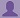SHARE
HELP

To start with, Sr2Jr’s first step is to reduce the expenses related to education. To achieve this goal Sr2Jr organized the textbook’s question and answers. Sr2Jr is community based and need your support to fill the question and answers. The question and answers posted will be available free of cost to all.# Absolute C++

Authors:
Walter Savitch ,kenrick Mock
Exercise:
Programming Projects
Chapter:
Constructors And Other Tools
Edition:
5
ISBN:
9780132846813
Question:
1
 Previous Next

###Question

Define a class called Month that is an abstract data type for a month. Your class will have one member variable of type int to represent a month (1 for January, 2 for February, and so forth). Include all the following member functions: a construc- tor to set the month using the first three letters in the name of the month as three arguments, a constructor to set the month using an integer as an argument (1 for January, 2 for February, and so forth), a default constructor, an input function that reads the month as an integer, an input function that reads the month as the first three letters in the name of the month, an output function that outputs the month as an integer, an output function that outputs the month as the first three letters in the name of the month, and a member function that returns the next month as a value of type Month . Embed your class definition in a test program.

###Answer

``````#include <iostream>
#include <stdio.h>
#include <stdlib.h>
using namespace std;

class Month
{
public:
Month(char m_firstLetter, char m_secondLetter, char m_thirdLetter);
Month(int monthInt);
Month();
void outputMonthInt();
void outputMonthLetters();
Month nextMonth();
private:
int month;
};

int main()
{
int monthInt;
char firstL, secondL, thirdL;
char test;
do {
cout << endl;

cout << "Testing the default constructor" << endl;
Month d;
d.outputMonthInt();
d.outputMonthLetters();
cout << endl;
cout << "Testing the constructor with one integer arguement" << endl;
cout << "Enter a month number: ";
cin >> monthInt;
if (monthInt <= 12 && monthInt >= 1)
{
Month num(monthInt);
num.outputMonthInt();
num.outputMonthLetters();
}
else
{
cout << "Invalid number for month, please choose from 1-12\n";
cout << "Enter a month number: ";
cin >> monthInt;
Month num(monthInt);
num.outputMonthInt();
num.outputMonthLetters();
}
cout << endl;
cout << "Testing the constructor with 3 letters as arguements" << endl;
cout << "Enter the first letter of a month in lowercase form: ";
cin >> firstL;
cout << "Enter the second letter of a month in lowercase form: ";
cin >> secondL;
cout << "Enter the third letter of a month in lowercase form: ";
cin >> thirdL;
cout << endl;
cout << "You have entered: " << firstL << secondL << thirdL << endl;

Month letter(firstL, secondL, thirdL);
letter.outputMonthLetters();
letter.outputMonthInt();
cout << endl;

cout << "Testing the member function that returns the next month as a value of type Month" << endl;
cin >> monthInt;
if (monthInt <= 12 && monthInt >= 1)
{
Month i(monthInt);
Month type = i.nextMonth();
cout << "Next Month" << endl;
type.outputMonthInt();
type.outputMonthLetters();
}
else
{
cout << "Invalid number for Month, choose between 1-12\n";
cout << "Enter a month nummber: ";
cin >> monthInt;
Month i(monthInt);
Month type = i.nextMonth();
cout << "Next Month" << endl;
type.outputMonthInt();
type.outputMonthLetters();
}
cout << endl;
cout << "Do you want to keep testing? y/n ";
cin >> test;
} while (test == 'y' || test == 'Y');
system("pause");
}

Month Month :: nextMonth()
{
if (month == 12)
return Month(1);
else
return Month(month + 1);
}

Month:: Month()
{
month = 1;
}
Month:: Month(int monthInt)
{
month = monthInt;
}

Month::Month(char first, char second, char third)
{
if ((first == 'j') && (second == 'a') && (third == 'n'))
month = 1;
if ((first == 'f') && (second == 'e') && (third == 'b'))
month = 2;
if ((first == 'm') && (second == 'a') && (third == 'r'))
month = 3;
if ((first == 'a') && (second == 'p') && (third == 'r'))
month = 4;
if ((first == 'm') && (second == 'a') && (third == 'y'))
month = 5;
if ((first == 'j') && (second == 'u') && (third == 'n'))
month = 6;
if ((first == 'j') && (second == 'u') && (third == 'l'))
month = 7;
if ((first == 'a') && (second == 'u') && (third == 'g'))
month = 8;
if ((first == 's') && (second == 'e') && (third == 'p'))
month = 9;
if ((first == 'o') && (second == 'c') && (third == 't'))
month = 10;
if ((first == 'n') && (second == 'o') && (third == 'v'))
month = 11;
if ((first == 'd') && (second == 'e') && (third == 'c'))
month = 12;
}
void Month :: outputMonthInt()
{
if (month >= 1 && month <= 12)
cout << "Month: " << month << endl;
else

cout << "Error - the month is not valid!" << endl;
}

void Month :: outputMonthLetters()
{
switch (month)
{
case 1:
cout << "Jan" << endl;
break;
case 2:
cout << "Feb" << endl;
break;
case 3:
cout << "Mar" << endl;
break;
case 4:
cout << "Apr" << endl;
break;
case 5:
cout << "May" << endl;
break;
case 6:
cout << "Jun" << endl;
break;
case 7:
cout << "Jul" << endl;
break;
case 8:
cout << "Aug" << endl;
break;
case 9:
cout << "Sep" << endl;
break;
case 10:
cout << "Oct" << endl;
break;
case 11:
cout << "Nov" << endl;
break;
case 12:
cout << "Dec" << endl;
break;
default:
cout << "Error - the month is not valid!" << endl;
}
} ``````

### Discussions

Post the discussion to improve the above solution.